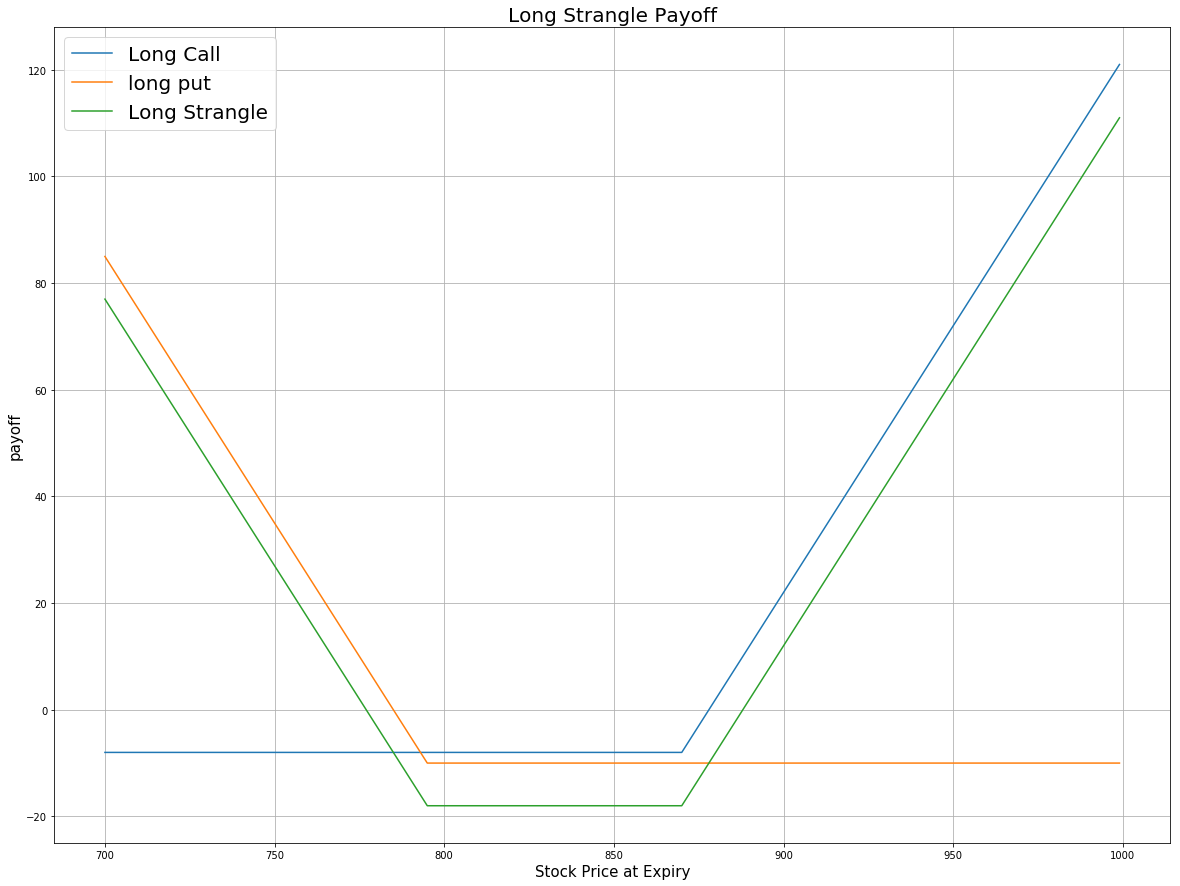### Definition

A Long Strangle is an options trading strategy that involves the simultaneous buying of an out-of-the-money put and an out-of-the-money call with the same underlying stock and expiration date. Similar to a Long Straddle, the Long Strangle has unlimited profit and limited risk, and can be applied if traders think the underlying asset will become volatile and move significantly in either direction. It differs from Long Straddle, however, in that the call strike is above the put strike.

price = np.arange(700,1000,1)
# Suppose the undelying price at time 0 is 830
k_call = 870 # The strike price of OTM call
k_put = 795 # The strike price of OTM put
# payoff for the long call
# payoff for the long put
payoff = np.sum([payoff_long_call, payoff_long_put], axis=0)
plt.figure(figsize=(20,15))
plt.plot(price, payoff_long_call, label = 'Long Call')
plt.plot(price, payoff_long_put, label = 'long put')
plt.plot(price, payoff, label = 'Long Strangle')
plt.legend(fontsize = 20)
plt.xlabel('Stock Price at Expiry',fontsize = 15)
plt.ylabel('payoff',fontsize = 15)
plt.title('Long Strangle Payoff',fontsize = 20)
plt.grid(True)From the payoff plot, the most that you can lose in a Long Strangle is the total premium you pay for holding the long position of both options. The maximum loss occurs when the stock price falls between the strike price of two options, in which case both options are worthless at expiration. The maximum gain of Long Strangle is unlimited for upside move because a stock's price has no maximum threshold.

### Implementation

Step 1: Initialize your algorithm including setting the start and end date, setting the cash and filtering the options contracts. Note here in SetFilter, the strike price should range from negative to positive because we need to choose out-of-the-money put and call options from candidate contracts. The strike price of OTM call should be greater than ATM options and the strike price of OTM put should be lower than ATM options.

def Initialize(self):
self.SetStartDate(2017, 4, 1)
self.SetEndDate(2017, 5, 30)
self.SetCash(100000)
self.symbol = option.Symbol
# set our strike/expiry filter for this option chain
option.SetFilter(-15, 15, timedelta(30), timedelta(60))
# use the underlying equity GOOG as the benchmark
self.SetBenchmark(equity.Symbol)


Step 2: Sort the option chain by expiration date and choose an expiration date you want to trade. For demonstration purpose, here we choose options with the furthest expiration date in candidate contracts. Then filter out the call options which expire on that date.

for i in optionchain:
if i.Key != self.symbol: continue
chain = i.Value
# sorted the option chain by expiration date and choose the furthest date
expiry = sorted(chain,key = lambda x: x.Expiry, reverse=True).Expiry
# filter the call options from the contracts expires on that date
call = [i for i in chain if i.Expiry == expiry and i.Right == 0]


Step 3: Sort the call options by their expiration date and choose the deep OTM contract which has the largest strike price.

call_contracts = sorted(call,key = lambda x: x.Strike)
if len(call_contracts) == 0: continue
# choose the deep OTM call option
self.call = call_contracts[-1]


Step 4: Select the put options which have the same expiration date with the call option and sort the put options by strike price. Then choose the deep out-of-the-money put which has the minimum strike price among all the available put options.

put_contracts = sorted([i for i in chain if i.Expiry == expiry and i.Right == 1], key = lambda x: x.Strike)
# choose the deep OTM put option
self.put = put_contracts


Step 5: Buy the call and the put options at the same time and wait until expiration.

self.Buy(self.call.Symbol ,1)


### Summary

In this algorithm, at time 0, we buy OTM call with strike price 870 and OTM put with strike price 795. The share price of GOOG at time 0 is $832.8. At the expiry, the share price of GOOG is$930.16, and so the call option is exercised and we get 100 shares of GOOG while the put option expires worthless.

You can enter into a Long Strangle if you have no clear idea of market direction but forecast that there will be a great movement in the underlying asset. As the options you buy are all out of the money, the premium cost of entering this position is low. However, because the call and the put options are all out of the money, the stock will need to move even more significantly than in a long straddle in order to profit from this strategy.

### Algorithm

Backtest using SetFilter

Backtest using OptionChainProvider